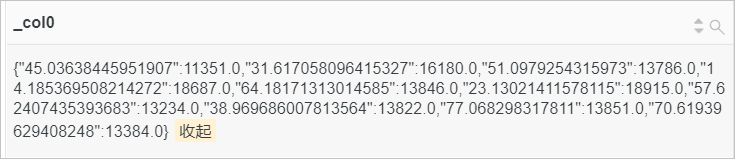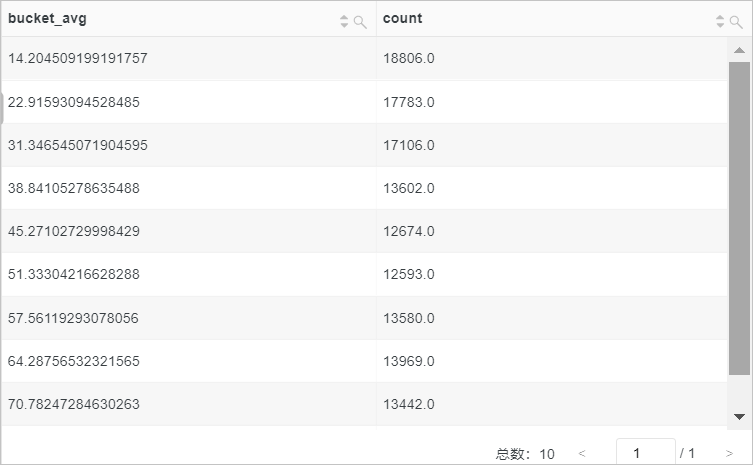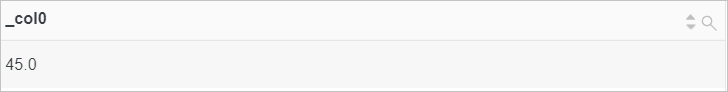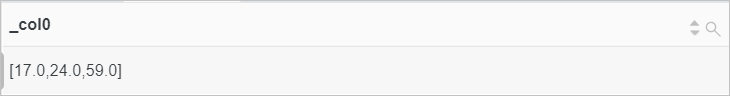approx_distinct函数 approx_distinct(key) 估算字段值中不重复值的个数，存在2.3%的标准误差。
numeric_histogram函数 numeric_histogram(bucket,key) 按照bucket数量（直方图列数），统计目标字段值的近似直方图。返回结果为JSON类型。
numeric_histogram_u函数 numeric_histogram_u(bucket,key) 按照bucket数量（直方图列数），统计目标字段值的近似直方图。返回结果为多行多列格式。
approx_percentile函数 approx_percentile(key,percentage) 对目标字段的值进行正序排列，返回大约处于percentage位置的值。
approx_percentile(key,array[percentage01,percentage02...]) 对目标字段的值进行正序排列，返回大约处于percentage01percentage02位置的值。

## approx_distinct函数

approx_distinct函数用于估算字段值中不重复值的个数，存在2.3%的标准误差。

### 语法

``approx_distinct(key)``

### 参数说明

key：字段名称，字段值可以为任意数据类型。

bigint类型。

### 示例

• 查询和分析语句
``* |SELECT count(*) AS PV, approx_distinct(client_ip) AS UV``
• 查询和分析结果## numeric_histogram函数

numeric_histogram函数按照bucket数量（直方图列数），统计目标字段值的近似直方图。返回结果为JSON类型。

### 语法

``numeric_histogram(bucket,key)``

### 参数说明

• bucket：直方图中列的个数，bigint类型。
• key：字段名称，double类型。

JSON类型。

### 示例

• 查询和分析语句
``request_method:POST | SELECT numeric_histogram(10,request_time)``
• 查询和分析结果## numeric_histogram_u函数

numeric_histogram_u函数按照bucket数量（直方图列数），统计目标字段值的近似直方图。返回结果为多行多列格式。

### 语法

``numeric_histogram_u(bucket,key)``

### 参数说明

• bucket：直方图中的列数，bigint类型。
• key：字段名称，double类型。

double类型。

### 示例

• 查询和分析语句
``request_method:POST | select numeric_histogram_u(10,request_time)``
• 查询和分析结果## approx_percentile函数

approx_percentile函数用于对目标字段的值进行正序排列，返回大约处于percentage位置的数值。

### 语法

• 返回处于percentage位置的数值，返回结果为double类型。
``approx_percentile(key,percentage)``
• 返回处于percentage01、percentage02位置的数值，返回结果为array类型。
``approx_percentile(key,array[percentage01,percentage02...])``

### 参数说明

• key：字段名称，字段值为bigint类型。
• percentage：百分比值，取值范围为[0,1]。

### 返回值类型

double类型或array类型。

### 示例

• 示例1：对request_time列进行排列后，返回大约处于50%位置的request_time字段的值。
• 查询和分析语句
``*| select approx_percentile(request_time,0.5)``
• 查询和分析结果• 示例2：对request_time列进行排列后，返回处于10%、20%及70%位置的request_time字段的值。
• 查询和分析语句
``*| select approx_percentile(request_time,array[0.1,0.2,0.7])``
• 查询和分析结果